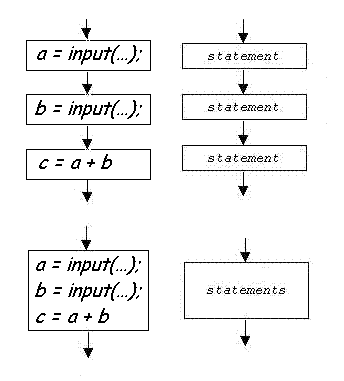﻿ ]>

## Sequential Execution

 A sequence is an ordered list of something. Sequential execution means that each command in a program script executes in the order in which it is listed in the program. The first command in the sequence executes first and when it is complete, the second command executes, and so on. Each of the four control flow diagram variations below, show graphically what sequential execution means. Multiple sequential statements are often shown as a single block of statements (as shown in two of the figures). For example, values are assigned to a and b before they are used to calculate the sum a+b. The following example is a sequence of commands that computes the average of three values. 1. Input the first value and save it as sum. 2. Input the second value and save it as nextValue. 3. Add nextValue to sum. 4. Input the third value and save it as nextValue. 5. Add nextValue to sum. 6. Divide sum by three and save the result as avg. 7. Display the average to the user. The order that the values are input and saved matter doesn't matter, but we can not calculate the average until all three values have been entered and added to together. The next step is to translate the sequential algorithm to code.